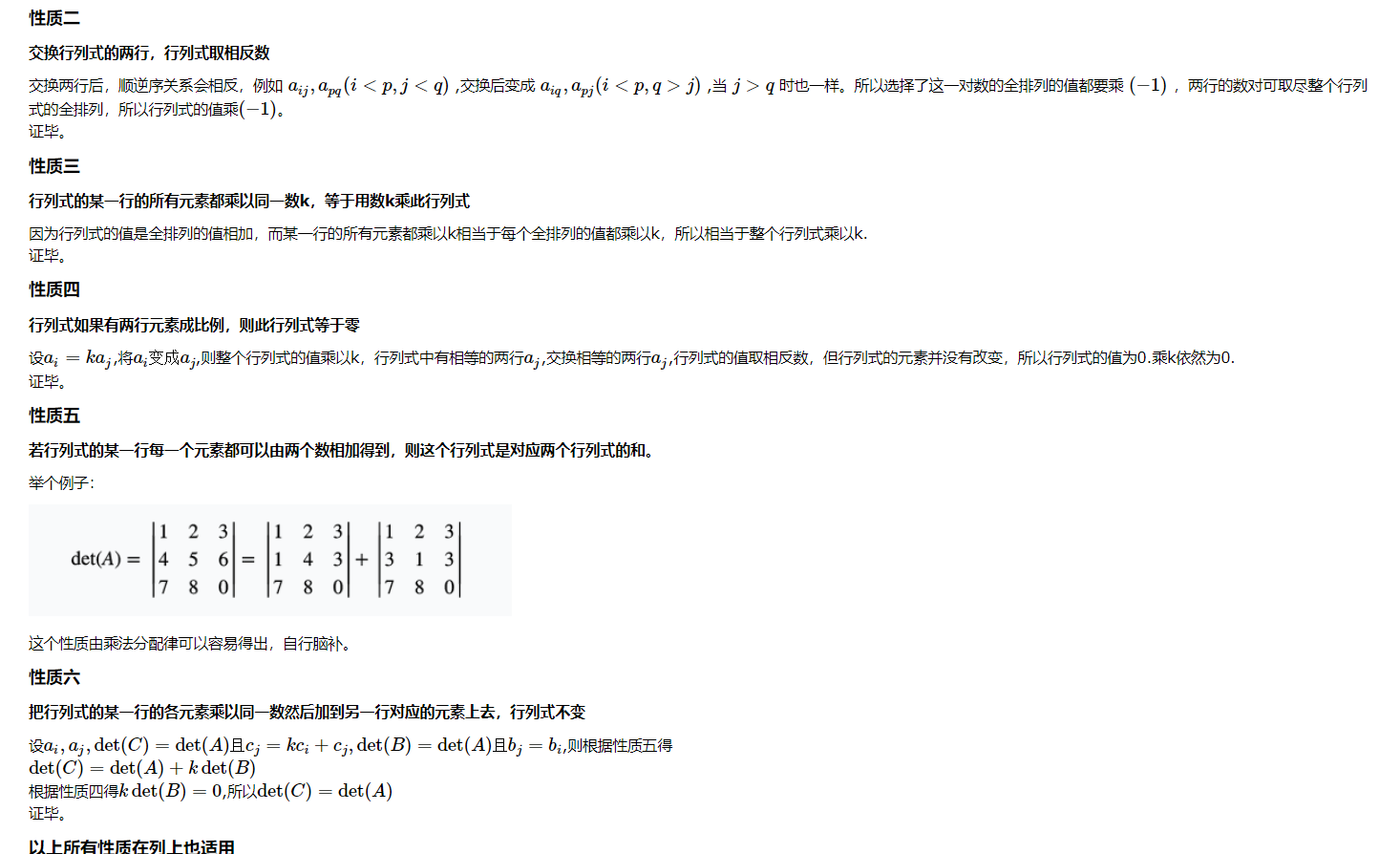XiShui's Blog 以梦为马，不负年华 2021-05-14T10:09:00.869Z http://krrrr.top/ 兮水XiShui丶 Hexo [数论][类欧几里德算法] http://krrrr.top/2021/05/14/数论-Meissel-Lehmer算法/ 2021-05-14T05:12:35.000Z 2021-05-14T10:09:00.869Z 类欧几里德算法由洪华敦在 2016 年冬令营营员交流中提出的内容，其本质可以理解为，使用一个类似辗转相除法来做函数求和的过程。

# 引入

]]>
<p>类欧几里德算法由洪华敦在 2016 年冬令营营员交流中提出的内容，其本质可以理解为，使用一个类似辗转相除法来做函数求和的过程。</p>

# 高斯消元

## 行列式求解思路

1、高斯消元交换两行时答案要除以$(−1)$.(性质二)
2、假设处理到第$i$个方程，一般的高斯消元是用第$i$个方程减去第$j(j>i$)个方程,所得的答案作为新的第$j$个方程，但求行列式的时候要用第$j$行减去第$i$行，所得答案作为新的第$j$行。因为方式一相当于某一行乘$(−1)$,另一行加到这一行上，这并不符合性质六。]]>
<p>学不会,什么都学不会呜呜/kk</p>
[思维][DP]CF1479B2 http://krrrr.top/2021/04/27/思维-DP-CF1479B2/ 2021-04-27T08:37:37.000Z 2021-04-27T09:31:13.389Z 呜呜呜

]]>
<p>呜呜呜</p>
[二分][思维]CF1479A http://krrrr.top/2021/04/27/二分-思维-CF1479A/ 2021-04-27T05:34:08.000Z 2021-04-27T05:55:04.626Z 呜呜

(nlog_2n)$## IDFT离散傅里叶反变换 使用快速傅里叶变换将多项式的点值表达转化为系数表达,这个过程叫做离散傅里叶反变换. 我们先设$(d_0,d_1,\dots,d_{n-1})$为$(a_0,a_1,\dots,a_{n-1})$进行$DFT$后的结果. 这时候我们构造一个多项式$F(x)=d_0+d_1x+\dots+d_{n-1}x^{n-1}$.然后我们再设向量$(c_0,c_1,\dots,c_{n-1})$为原多项式进行$DFT$后的点值表示.其中$c_k$表示$F(x)$在$x=\omega_n^{-k}$的点值表示. 即$c_k=\sum_{i=0}^{n-1}d_i(\omega_n^{-k})^i$然后我们考虑把$d_i$分解,用$a_i$表示,我们得到了 然后我们把$a_j$扔到外面,就得到了 然后我们把$(\omega_n^{-k})^i$转换为$(\omega_n^i)^{-k}$并把最后两项合并之后有 然后我们把$\sum_{i=0}^{n-1}(\omega_n^i)^{j-k}$单独拿出来,并且设$\xi=j-k$,然后我们发现这个柿子就等于 我们观察一下这个柿子,发现我们可以用等比数列求和公式来直接求出这个柿子的值,而且这个柿子恰好等于$0$. 那么原来的柿子就可以这样乱搞: 对于多项式$A(x)$由插值节点$\left(\omega_{n}^{0}, \omega_{n}^{1}, \omega_{n}^{2}, \ldots, \omega_{n}^{n-1}\right)$做离散傅里叶变换得到的点值向量$\left(d_{0}, d_{1}, \ldots, d_{n-1}\right)$。我们将$\left(\omega_{n}^{0}, \omega_{n}^{-1}, \omega_{n}^{-2}, \ldots, \omega_{n}^{-(n-1)}\right)$作为插值节点,$\left(d_{0}, d_{1}, \ldots, d_{n-1}\right)$作为系数向量, 做一次离散傅里叶变换得到的向量每一项都除以$n$之后得 到的$\left(\frac{c_{0}}{n}, \frac{c_{1}}{n}, \ldots, \frac{c_{n-1}}{n}\right)$就是多项式的系数向量$\left(a_{0}, a_{1}, \ldots, a_{n-1}\right)$。 注意到$\omega_{n}^{-k}$是$\omega_{n}^{k}$的共轭复数。 ## FFT朴素版代码 ## 迭代实现 先盗一下图(我们观察一下原序列和后序列,然后我们发现了一个显然的的结论: 我们需要求的序列实际是原序列下标的二进制反转！ 因此我们对序列按照下标的奇偶性分类的过程其实是没有必要的,所以这样我们可以$O(n)$的利用某种操作得到我们要求的序列，然后不断向上合并就好了 ## FFT代码(迭代实现) # 快速数论变换(NTT) 因为知道了自己是多么的菜,所以才要更加努力去追求那个永远也不可能实现的梦想 ]]> <p>开始跳楼了</p> 博弈论训练 http://krrrr.top/2020/10/22/博弈论训练/ 2020-10-22T04:12:33.000Z 2020-11-02T08:12:56.992Z 感觉更多的还是要靠思维…. 因为知道了自己是多么的菜,所以才要更加努力去追求那个永远也不可能实现的梦想 ]]> <p>感觉更多的还是要靠思维….<br> 数分训练 http://krrrr.top/2020/10/19/数分训练/ 2020-10-19T13:15:44.000Z 2020-10-19T13:29:37.180Z 啥都不会啊QAQ # 1.实数集与函数 ## 1.实数 ### 1.1.1 设$a,b,c \in R^+$,证明$|\sqrt{a^2+b^2} - \sqrt{a^2+c^2}| \leq |b - c|$证明:首先两边同时乘以$|\sqrt{a^2+b^2}+\sqrt{a^2+c^2}|$后有 化简之后可以得到 又$|b + c| \leq |b| + |c|$,则只需证明$|b| + |c| \leq |\sqrt{a^2+b^2}+\sqrt{a^2+c^2}|$又$\sqrt{x}$显然大于$0$,则$\sqrt{a^2+b^2}$显然大于$|b|$,同理,$\sqrt{a^2+c^2}$显然大于$|c|$得证.$QED$下面我们来讨论这个柿子的几何意义 我们将这个柿子做一个简简单单的变换 即这个柿子可以看成点$(a,b)$到原点的距离,同理,后边的那个柿子可以看成点$(a,c)$到原点的距离.那么那个大柿子就可以看作两点到原点距离之差,而我们又发现这两点之间的距离就等于$|b-c|$.而这两个点与原点又可以构成三角形.所以几何意义显然 三角形两边之差小于第三边. ### 1.1.2 不会了xd 因为知道了自己是多么的菜,所以才要更加努力去追求那个永远也不可能实现的梦想 ]]> <p>啥都不会啊QAQ<br> ABC178 http://krrrr.top/2020/10/09/ABC178/ 2020-10-09T11:18:59.000Z 2020-10-09T11:32:34.804Z ABC只会E人从来都是笑着面对生活 D.Redistribution 因为知道了自己是多么的菜,所以才要更加努力去追求那个永远也不可能实现的梦想 ]]> <p>ABC只会E人从来都是笑着面对生活</p> ABC179 http://krrrr.top/2020/10/08/ARC179/ 2020-10-08T04:36:17.000Z 2020-10-09T10:53:14.138Z 既然是ABC就只看DEF了…. D.- Leaping Tak 我们用推的方法可以发现我们对于每个位置$i$,可以枚举每个$i$能到的位置然后$dp$转移. 然后发现对于每次转移,等价与将$k$段连续区间的$dp$值加一个相同的数.然后我们最后要的就是$dp_n$. 对于这种区间修改单点求值的问题我们显然可以用树状数组来维护. E.Sequence Sum 我们发现$n$的值很大,所以不能直接去算.但是我们发现$M$的值只有$1e5$的级别.所以我们由抽屉原理可知,我们至多只需要枚举$m+1$个数字,就一定可以找到一个和之前重复的数字,而假设我们现在枚举到的重复的位置为$j$,这个数字之前出现过的位置为$i$,那么剩余的数字一定都是位置$i$到位置$j$的数字的重复. F. Simplified Reversi 发现$n$和$q$都是$2 \times 10^5$级别,所以直接模拟显然不太核里…发现每一次操作都最多只会删掉一行最靠左上的部分.我们设现在的最左上方的长宽分别为$c$和$k$. 刚开始的时候$c=k=n-2$,我们进行任何一次操作之后黑色棋子的个数都会减掉$n-2$.假设我们进行了$1$操作在坐标为$(1,xx)$的位置,那么我们在(1,xx)以后的位置再进行一次一操作的话,黑色棋子的个数都一定会只减少$n-2$. 所以我们对于列的情况,对于刚开始的$n-2$列,我们把这$n-2$列的值都设为$n-2$,表示对这$n-2$列操作时,都会将黑色棋子的个数减少$$n-2$.然后在进行某一次$2$操作时,假设进行的$2$操作是第$yy$行,那么我们发现,从第$yy$行往下的所有白棋子都不会再被$1$操作所染白. 而从左到第一个$1$操作的所有列上再进行$1$操作都会只染白$[2,yy)$这个区间的棋子

]]>
<p>既然是ABC就只看DEF了….</p>
209191110模拟赛题解 http://krrrr.top/2019/11/11/209191110模拟赛题解/ 2019-11-10T23:33:11.000Z 2019-11-11T03:07:03.159Z $FPX$牛逼!

### kyouko

$70$分做法:我们发现我们可以枚举每个数字,然后统计这个数字的$i$次方.然后随便开个$set$去个重之类的就$70$了.

### chisa

]]>
<p>$FPX$牛逼!</p>

### 最优连通子集20年前的Noi题目

### The more, The Better### 选课

]]>
<p>我真的菜死了啊/(ㄒoㄒ)/~~<br>

### 打饭

]]>
<p>版权原因,题面不公开.<br>
20191107模拟赛题解 http://krrrr.top/2019/11/08/20191107模拟赛题解/ 2019-11-08T00:25:20.000Z 2019-11-09T00:49:47.912Z 德州扑克真好玩.jpg

### 多边形

#### 思路

]]>
<p>德州扑克真好玩.jpg<br>
20191106模拟赛 http://krrrr.top/2019/11/07/20191106模拟赛/ 2019-11-07T01:13:28.000Z 2019-11-07T02:50:13.559Z 给我也整一个这样的评测环境### B

#### 思路

• 可行性剪枝:一旦超过$3000$就剪掉
• 去重:例如$2,3,2,3,4,5$和$2,2,3,3,4,5$是相似的指数不同的排列所以我们搜的时候只搜后面的情况然后通过组合数直接算出来就行了
• 预处理:我们发现每次的搜索过程都是一样的只是$n$不同 我们现在搜一次将答案存到桶里每次询问直接输出就行了

]]>